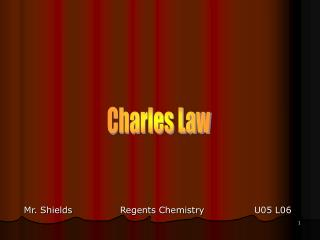DownloadDownload PresentationCharles Law

# Charles Law

Download Presentation## Charles Law

- - - - - - - - - - - - - - - - - - - - - - - - - - - E N D - - - - - - - - - - - - - - - - - - - - - - - - - - -
##### Presentation Transcript

1. Charles Law Mr. Shields Regents Chemistry U05 L06

2. Charles Law V/T = k (constant P and n) • Jacques Charles (1746 – 1823) • Charle’s Law (1787) • Volume vs. Temp relationshipof a gas

3. Charles Law • In 1787 Charle’s law was published • This law looked at the variables of Volume (V) and • Temperature (T) • V/T = k V, T = variables P, n = Constants Charles’s law states that the volume of a gas at constant p,n varies DIRECTLY with it’s absolute Temperature (in Kelvin!)

4. Developing Charles Law Since velocity will increases with increasing temp then The number of collisions with the containers wall per unit time must be increasing And increasing the rate of collisions with the containers Walls increases pressure BUT Charles law assumes constant P with increasing temp. So how does P stay constant if T increases ?

5. Developing Charles Law Remember Boyles law? Boyles law says we can reduce the pressure if we Increase the volume PV=k As V P In other words, if there is a driving force that increases pressure we can decrease the pressure by increasing the container volume.

6. Developing Charles Law So if T increases we can hold pressure constant if we Increase volume at the same time T increases This is just what Charles Law says V/T = k A direct relationship (p,n const) Or in a more useful form for calculations: V1/T1 = V2/T2 (why is this true?) So what does the relationship V/T look like?

7. Note: Plot relates deg C to V but Calculations were fist done in deg. K Charles Law Below is a data table relating temperature to volume Note that all V/T equals the same k (try trial 2,4,8) A graph of this data looks like this:

8. Why Kelvin for Calculations? • T and V are related such that for each doubling • (or Halving) of the temperature (in Kelvin) Volume • Doubles (or halves) i.e a direct relationship • NOTE WELL: This relationship is only true for Deg. • KelvinNOT degrees Celsius. But Why? • What would happen if the temp were 0 deg. Celsius • Division by zero is undefined 2x 1x 100k 200k

9. Why Kelvin? • And What would happen if Temperature was NEAGATIVE? • Predicted Volume would be negative • Let’s try it: • If V1=1 L and T1 = 25 deg C and T2 equals –50 deg C • what does V2 equal? • V1/T1=V2/T2; 1/25 = V2/-50 Then V2 = -2 L (????)

10. Decreasing Temperatures • What happens when a gas drops to VERY LOW TEMP • (assuming the gas does not condense to a liquid)? • Charles law would predict the following: • The direct relationship of V/T remains a straight line • (2) Extending the line to zero volume shows there • is a lower limit to temperature (i.e. Avg KE) • The lower limit for temperature is 0 deg. Kelvin. • This is called ABSOLUTE ZERO

11. T = 343 K T = 171.5 K 0 K There is a direct relationship between T(kelvin), V and E There is a Direct Relationship Between Tkelvin And Energy Earths coldest Recorded Temp: -132 deg C High Antartic Polar Plateau E

12. 2 atm 1 atm 0.5 atm Assumes gases do not Condense to a liquid Absolute zero: -273 deg C or 0 K V vs. T at Different P V/T = k (p,n held const.) No matter what the initial P is all lines intersect at Absolute zero

13. Let’s try a few problems.

14. Problem 1 & 2 If the temperature of the gas in fig.1 is 35 deg. C and the volume is 1/2L what is the temperature in fig.2 and What is the temp in fig 3? (P,n are constant) Fig 1 Fig 2 Fig. 3 V1/T1 = V2/T2 .5L/308 = 1L/T2 T2 = 1L/(.5L/308) T2 = 616 deg K V1/T1 = V3/T3 .5L/308=2L/T3 T3 = 2L/(.5L/308) T3 = 1232 deg. K ½ L 1 L 2L

15. Problem 3 A sample of gas at 30 deg C and standard pressure (1 atm) occupies a volume of 200 ml. Calculate the temperature in deg. Celsius at which the gas will occupy a volume of 5 ml at std pressure if P is held constant. V1/T1 = V2/T2 T2 = V2/(V1/T1) T2 = 5/(200/303) T2 = 7.58 deg K What’s this temperature in deg. C? T2 = -265.42 deg. C (K = C + 273)